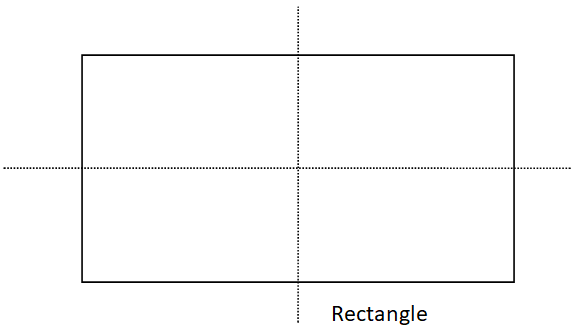Courses
Courses for Kids
Free study material
Free LIVE classes
More# How many lines of symmetry does a rectangle have?$A.{\text{ }}1 \\ B.{\text{ 2}} \\ C.{\text{ 3}} \\ D.{\text{ 4}} \\$Verified
328.2k+ views
Hint- We will find out the number of lines that can be drawn across the rectangle such that we obtain an exact mirror image every time with the help of a figure.$\Rightarrow$ There are $2$ lines of symmetry of a rectangle which are from the midpoints of the length and the breadth of the rectangle.
$\Rightarrow$ These are two lines as shown in the figure that cut the rectangle in two similar halves which are mirror images of each other. If a rectangle is folded along its line of symmetry, it superimposes perfectly.
Hence, Rectangle has $2$ lines of symmetry.